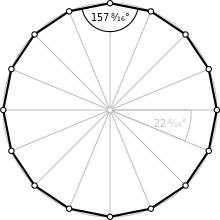Type Regular polygon
Edges and vertices 16
Schläfli symbol {16}, t{8}, tt{4}
Coxeter diagram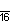Symmetry group Dihedral (D16), order 2×16
Internal angle (degrees) 157.5°
Dual polygon Self
Properties Convex, cyclic, equilateral, isogonal, isotoxal

In mathematics, a hexadecagon (sometimes called a hexakaidecagon) or 16-gon is a sixteen-sided polygon.

A regular hexadecagon is a hexadecagon in which all angles are equal and all sides are congruent. Its Schläfli symbol is {16} and can be constructed as a truncated octagon, t{8}, and a twice-truncated square tt{4}. A truncated hexadecagon, t{16}, is a triacontadigon, {32}.

### Construction

As 16 = 24 (a power of two), a regular hexadecagon is constructible using compass and straightedge: this was already known to ancient Greek mathematicians.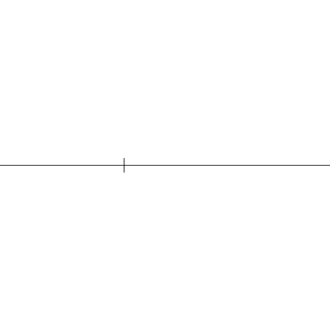at a given circumcircle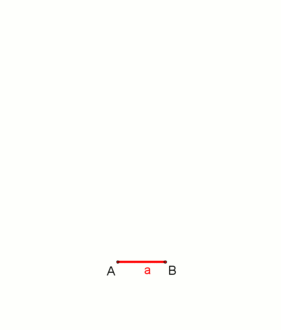at a given side length, animation. (The construction is very similar to that of octagon at a given side length.)

## Measurements

Each angle of a regular hexadecagon is 157.5 degrees, and the total angle measure of any hexadecagon is 2520 degrees.

The area of a regular hexadecagon with edge length t isBecause the hexadecagon has a number of sides that is a power of two, its area can be computed in terms of the circumradius R by truncating Viète's formula:Since the area of the circumcircle isthe regular hexadecagon fills approximately 97.45% of its circumcircle.

## Symmetry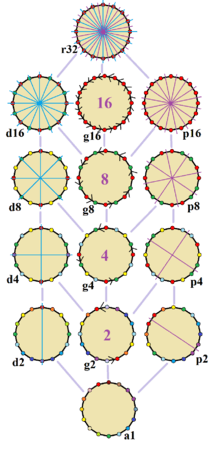The 14 symmetries of a regular hexadecagon. Lines of reflections are blue through vertices, purple through edges, and gyration orders are given in the center. Vertices are colored by their symmetry position.

The regular hexadecagon has Dih16 symmetry, order 32. There are 4 dihedral subgroups: Dih8, Dih4, Dih2, and Dih1, and 5 cyclic subgroups: Z16, Z8, Z4, Z2, and Z1, the last implying no symmetry.

On the regular hexadecagon, there are 14 distinct symmetries. John Conway labels full symmetry as r32 and no symmetry is labeled a1. The dihedral symmetries are divided depending on whether they pass through vertices (d for diagonal) or edges (p for perpendiculars) Cyclic symmetries in the middle column are labeled as g for their central gyration orders.

The most common high symmetry hexadecagons are d16, a isogonal hexadecagon constructed by eight mirrors can alternate long and short edges, and p16, an isotoxal hexadecagon constructed with equal edge lengths, but vertices alternating two different internal angles. These two forms are duals of each other and have half the symmetry order of the regular hexadecagon.

Each subgroup symmetry allows one or more degrees of freedom for irregular forms. Only the g16 subgroup has no degrees of freedom but can seen as directed edges.

{8}#{ } { 83 }#{ } { 85 }#{ }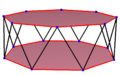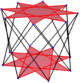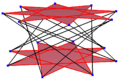A regular skew hexadecagon is seen as zig-zagging edges of a octagonal antiprism, a octagrammic antiprism, and a octagrammic crossed-antiprism.

A skew hexadecagon is a skew polygon with 24 vertices and edges but not existing on the same plane. The interior of such an hexadecagon is not generally defined. A skew zig-zag hexadecagon has vertices alternating between two parallel planes.

A regular skew hexadecagon is vertex-transitive with equal edge lengths. In 3-dimensions it will be a zig-zag skew hexadecagon and can be seen in the vertices and side edges of a octagonal antiprism with the same D8d, [2+,16] symmetry, order 32. The octagrammic antiprism, s{2,16/3} and octagrammic crossed-antiprism, s{2,16/5} also have regular skew octagons.

### Petrie polygons

The regular hexadecagon is the Petrie polygon for many higher-dimensional polytopes, shown in these skew orthogonal projections, including:

A15 B8 D9 2B2 (4D)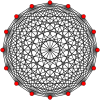15-simplex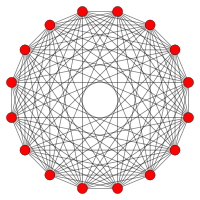8-orthoplex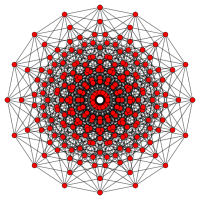8-cube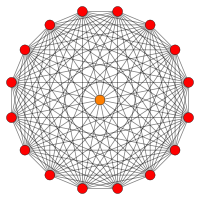611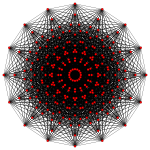161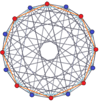8-8 duopyramid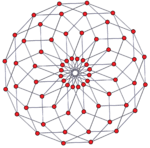8-8 duoprism

## Related figures

A hexadecagram is a 16-sided star polygon, represented by symbol {16/n}. There are three regular star polygons, {16/3}, {16/5}, {16/7}, using the same vertices, but connecting every third, fifth or seventh points. There are also three compounds: {16/2} is reduced to 2{8} as two octagons, {16/4} is reduced to 4{4} as four squares and {16/6} reduces to 2{8/3} as two octagrams, and finally {16/8} is reduced to 8{2} as eight digons.

Deeper truncations of the regular octagon and octagram can produce isogonal (vertex-transitive) intermediate hexadecagram forms with equally spaced vertices and two edge lengths.

A truncated octagon is a hexadecagon, t{8}={16}. A quasitruncated octagon, inverted as {8/7}, is a hexadecagram: t{8/7}={16/7}. A truncated octagram {8/3} is a hexadecagram: t{8/3}={16/3} and a quasitruncated octagram, inverted as {8/5}, is a hexadecagram: t{8/5}={16/5}.

## In art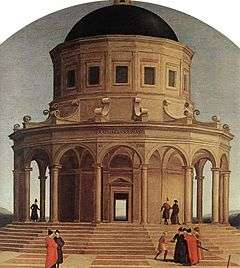The hexadecagonal tower from Raphael's The Marriage of the Virgin

In the early 16th century, Raphael was the first to construct a perspective image of a regular hexadecagon: the tower in his painting The Marriage of the Virgin has 16 sides, elaborating on an eight-sided tower in a previous painting by Pietro Perugino.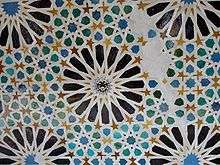A hexadecagrammic pattern from the Alhambra

Hexadecagrams (16-sided star polygons) are included in the Girih patterns in the Alhambra.

An octagonal star can be seen as a concave hexadecagon: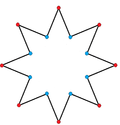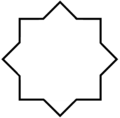## References

1. Weisstein, Eric W. (2002). CRC Concise Encyclopedia of Mathematics, Second Edition. CRC Press. p. 1365. ISBN 9781420035223.
2. Koshy, Thomas (2007), Elementary Number Theory with Applications (2nd ed.), Academic Press, p. 142, ISBN 9780080547091.
3. John H. Conway, Heidi Burgiel, Chaim Goodman-Strauss, (2008) The Symmetries of Things, ISBN 978-1-56881-220-5 (Chapter 20, Generalized Schaefli symbols, Types of symmetry of a polygon pp. 275-278)
4. The Lighter Side of Mathematics: Proceedings of the Eugène Strens Memorial Conference on Recreational Mathematics and its History, (1994), Metamorphoses of polygons, Branko Grünbaum
5. Speiser, David (2011), "Architecture, mathematics and theology in Raphael's paintings", in Williams, Kim, Crossroads: History of Science, History of Art. Essays by David Speiser, vol. II, Springer, pp. 29–39, doi:10.1007/978-3-0348-0139-3_3. Originally published in Nexus III: Architecture and Mathematics, Kim Williams, ed. (Ospedaletto, Pisa: Pacini Editore, 2000), pp. 147–156.
6. Hankin, E. Hanbury (May 1925), "Examples of methods of drawing geometrical arabesque patterns", The Mathematical Gazette, 12 (176): 370–373, doi:10.2307/3604213.